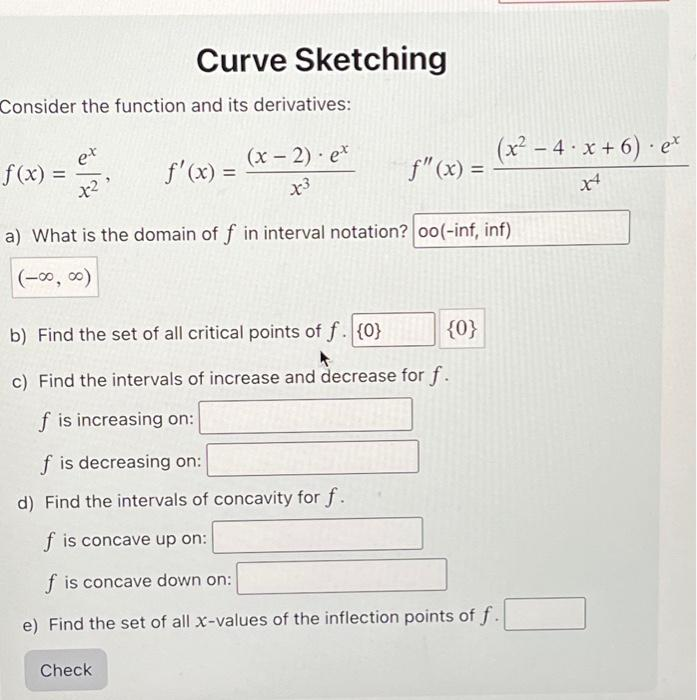Home / Expert Answers / Calculus / consider-the-function-and-its-derivatives-f-x-ex-x-curve-sketching-3-check-f-39-x-x-2-ex-pa156

# (Solved): Consider the function and its derivatives: f(x) = ex x Curve Sketching 3 Check f'(x) = (x - 2). ex ...

Consider the function and its derivatives: f(x) = ex x² Curve Sketching 3 Check f'(x) = (x - 2). ex x3 f" (x) = (x² - 4x+6) · ex X4 a) What is the domain of f in interval notation? oo(-inf, inf) (-?, ?) b) Find the set of all critical points of f. {0} {0} c) Find the intervals of increase and decrease for f. f is increasing on: f is decreasing on: d) Find the intervals of concavity for f. f is concave up on: f is concave down on: e) Find the set of all x-values of the inflection points of f.Consider the function and its derivatives: a) What is the domain of in interval notation? b) Find the set of all critical points of . c) Find the intervals of increase and decrease for . is increasing on: is decreasing on: d) Find the intervals of concavity for . is concave up on: is concave down on: e) Find the set of all -values of the inflection points of .

We have an Answer from Expert